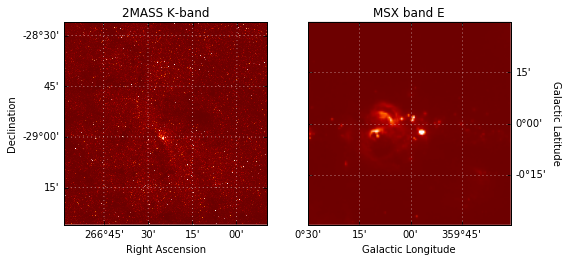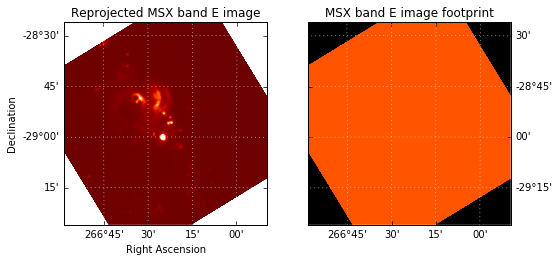# Affiliated package: reproject¶

The reproject package can be found here. To install it:

pip install reproject

The reproject package implements image reprojection (resampling) methods for astronomical images and more generally n-dimensional data. These assume that the WCS information contained in the data are correct. This package does not do image registration, which is the process of aligning images where one or more images may have incorrect or missing WCS.

In :
from astropy.io import fits
from astropy.utils.data import get_pkg_data_filename

In :
hdu1 = fits.open(get_pkg_data_filename('galactic_center/gc_2mass_k.fits'))
hdu2 = fits.open(get_pkg_data_filename('galactic_center/gc_msx_e.fits'))

In :
from astropy.wcs import WCS
%matplotlib inline
import matplotlib.pyplot as plt

fig = plt.figure(figsize=(8,6))

ax1.imshow(hdu1.data, origin='lower',
vmin=-100., vmax=2000.,
cmap=plt.cm.gist_heat)

ax1.coords.grid(color='white')
ax1.coords['ra'].set_axislabel('Right Ascension')
ax1.coords['dec'].set_axislabel('Declination')
ax1.set_title('2MASS K-band')

ax2.imshow(hdu2.data, origin='lower',
vmin=-2.e-4, vmax=5.e-4,
cmap=plt.cm.gist_heat)

ax2.coords.grid(color='white')
ax2.coords['glon'].set_axislabel('Galactic Longitude')
ax2.coords['glat'].set_axislabel('Galactic Latitude')
ax2.coords['glat'].set_axislabel_position('r')
ax2.coords['glat'].set_ticklabel_position('r')
ax2.set_title('MSX band E')

Out:
<matplotlib.text.Text at 0x11207bc88>We now reproject the MSX image to be in the same projection as the 2MASS image:

In :
from reproject import reproject_interp


The reproject_interp() function above returns the reprojected array as well as an array that provides information on the footprint of the first image in the new reprojected image plane (essentially which pixels in the new image had a corresponding pixel in the old image). We can now visualize the reprojected data and footprint:

In :
fig = plt.figure(figsize=(8,6))

ax1.imshow(array, origin='lower',
vmin=-2.e-4, vmax=5.e-4,
cmap=plt.cm.gist_heat)
ax1.coords.grid(color='white')
ax1.coords['ra'].set_axislabel('Right Ascension')
ax1.coords['dec'].set_axislabel('Declination')
ax1.set_title('Reprojected MSX band E image')

ax2.imshow(footprint, origin='lower',
vmin=0, vmax=1.5,
cmap=plt.cm.gist_heat)
ax2.coords.grid(color='white')
ax1.coords['ra'].set_axislabel('Right Ascension')
ax1.coords['dec'].set_axislabel('Declination')
ax2.coords['dec'].set_axislabel_position('r')
ax2.coords['dec'].set_ticklabel_position('r')
ax2.set_title('MSX band E image footprint')

Out:
<matplotlib.text.Text at 0x11355d898>We can then write out the image to a new FITS file. Note that, as for plotting, we can use the header from the 2MASS image since both images are now in the same projection:

In :
fits.writeto('msx_on_2mass_header.fits', array, hdu1.header, clobber=True)


## Pratical Exercise¶

Try and reproject some of your images if you have any! Otherwise, you can check the documentation for reproject to see what other options/algorithms are available.